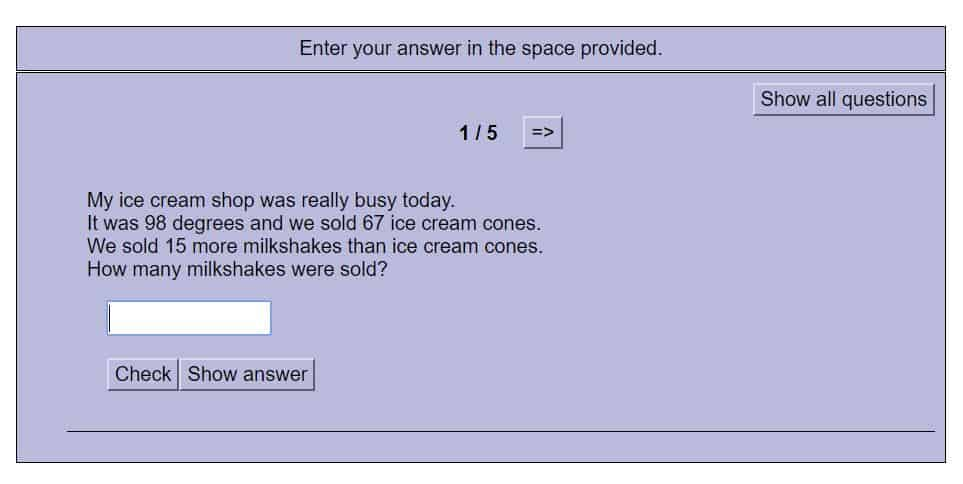# Problem Solving – Thinking Blocks Interactive Tutorials and Problems 3

Thinking Blocks Interactive Tutorials – Model and solve word problems with addition and subtraction.  Online interactive tutorial (First-Fourth).

#### Online Word Problems with Addition and Subtraction Challenge Problems#### Represent and solve problems involving addition and subtraction.

CCSS.MATH.CONTENT.2.OA.A.1
Use addition and subtraction within 100 to solve one- and two-step word problems involving situations of adding to, taking from, putting together, taking apart, and comparing, with unknowns in all positions, e.g., by using drawings and equations with a symbol for the unknown number to represent the problem.

#### Solve problems involving the four operations, and identify and explain patterns in arithmetic.

CCSS.MATH.CONTENT.3.OA.D.8
Solve two-step word problems using the four operations. Represent these problems using equations with a letter standing for the unknown quantity. Assess the reasonableness of answers using mental computation and estimation strategies including rounding.

#### Use the four operations with whole numbers to solve problems.

CCSS.MATH.CONTENT.4.OA.A.3
Solve multistep word problems posed with whole numbers and having whole-number answers using the four operations, including problems in which remainders must be interpreted. Represent these problems using equations with a letter standing for the unknown quantity. Assess the reasonableness of answers using mental computation and estimation strategies including rounding.